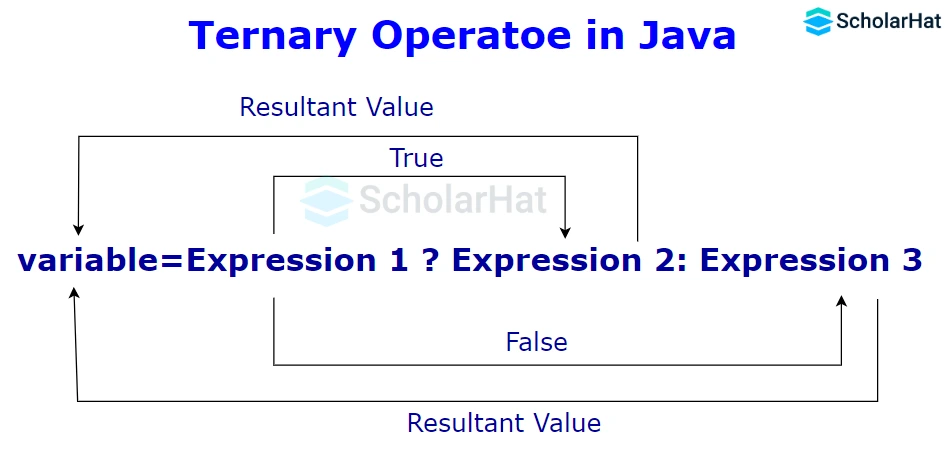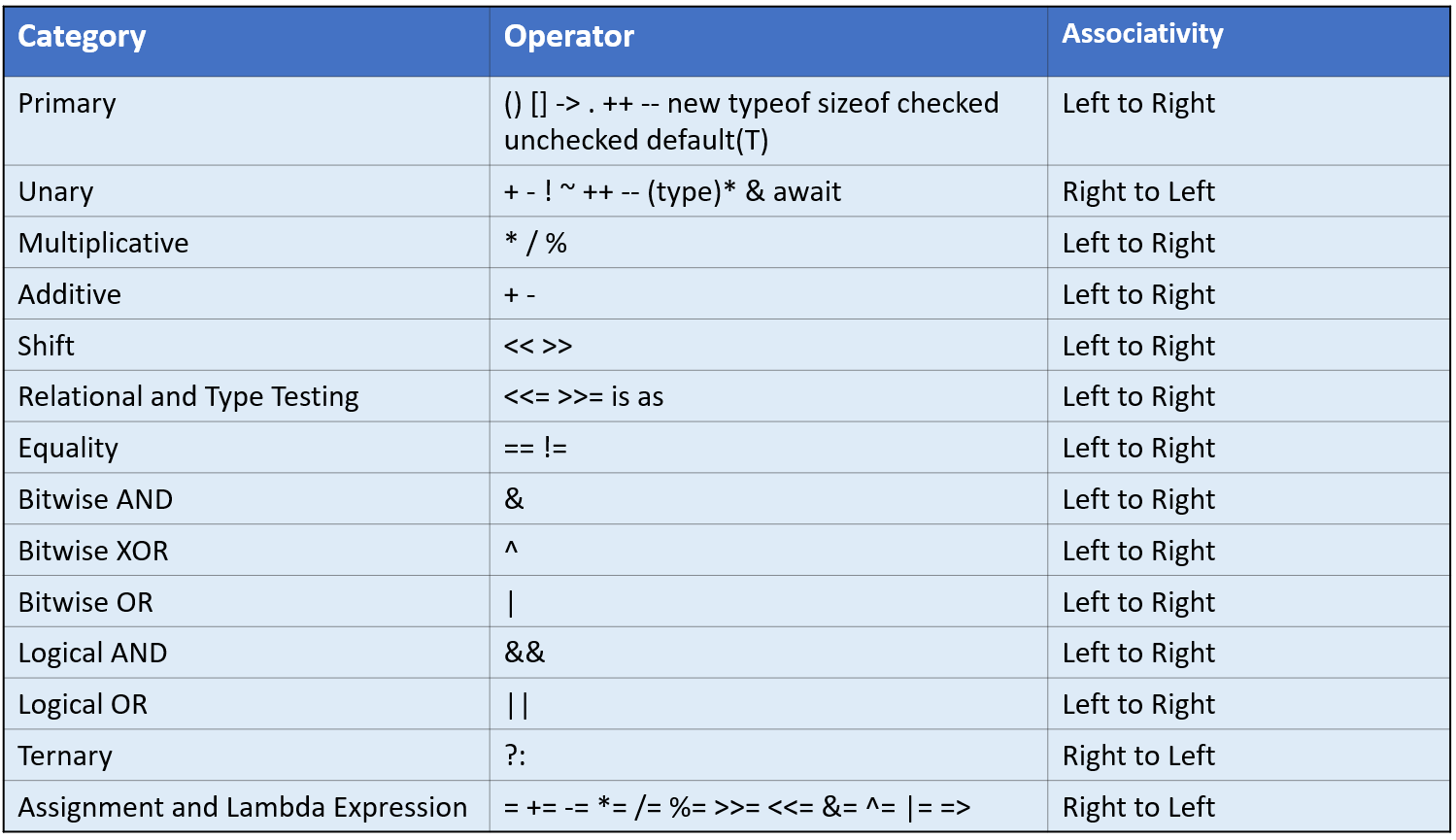# Operators in Java: Arithmetic, Relational, Logical, and more

22 Sep 2023
Beginner
915 Views

## Operators in Java: An Overview

Are you a learner looking to Learn Java with a comprehensive guide on Java Operators? Look no further! In this article, we'll explore the different types of operators available in Java and give examples of how they can be used. We'll cover unary and binary operators, assignment and comparison operators, java logical operators, increment/decrement operations, bitwise operations, Java Operators, and Java operators examples much more - so get your programmer's hat on, and let’s dig into some cool coding with operands!

## What are Java Operators?

Operators in Java, a Java toolkit, are being used as a symbol that performs various operations according to the code. Some Operators of JAVA are "+","-","*","/" etc. The idea of using Operators has been taken from other languages so that it behaves expectedly.

## Types of Operators in Java

There are various types of Operators in Java that are used for operating. These are,

1. Arithmetic operators in Java
2. Relational operators in Java
3. Logical operators in Java
4. Assignment operator in Java
5. Unary operator in Java
6. Bitwise operator in Java
7. Comparison operator in Java
8. Ternary operator in Java### Arithmetic Operators in Java

Arithmetic Operators in Java are particularly used for performing arithmetic operations on given data or variables. There are various types of operators in Java, such as

Operators Operations
- Subtraction
x Multiplication
/ Division
% Modulus

#### Example

``````class Main
{
public static void main(String[] args)
{

// declare variables
int a = 15, b = 5;

System.out.println("a + b = " + (a + b));

// subtraction operator
System.out.println("a - b = " + (a - b));

// multiplication operator
System.out.println("a * b = " + (a * b));

// division operator
System.out.println("a / b = " + (a / b));

// modulo operator
System.out.println("a % b = " + (a % b));

}
}
``````

#### Output

``````a + b = 20a - b = 10a × b = 75
a ÷ b = 3
a % b = 7``````

### Assignment Operator in Java

Assignment Operators are mainly used to assign the values to the variable that is situated in Java programming. There are various assignment operators in Java, such as

Operators Examples Equivalent to
= X = Y; X = Y;
+= X += Y; X = X + Y;
-= X -= Y; X = X - Y;
*= X *= Y; X = X * Y;
/= X /= Y; X = X / Y;
%= X %= Y; X = X % Y;

#### Example

``````class Main
{
public static void main(String[] args)
{

// create variables
int a = 5;
int var;

// assign value using =
var = a;

System.out.println("var using =: " + var);

// assign value using =+
var += a;

System.out.println("var using +=: " + var);

// assign value using =*
var *= a;

System.out.println("var using *=: " + var);

}
}
``````

#### Output

``````var using =: 5
var using +=: 10
var using *=: 50``````

### Relational Operators in Java

Java relational operators are assigned to check the relationships between two particular operators. There are various relational operators in Java, such as

Operators Description Example
== Is equal to 3 == 5 returns false
!= Not equal to 3 != 5 returns true
> Greater than 3 > 5 returns false
< Less than 3 < 5 returns true
>= Greater than or equal to 3 >= 5 returns false
<= Less than or equal to 3 <= 5 returns true

#### Example

``````class Main
{
public static void main(String[] args)
{

// create variables
int a = 7, b = 11;

// value of a and b
System.out.println("a is " + a + " and b is " + b);

// == operator
System.out.println(a == b); // false

// != operator
System.out.println(a != b); // true

// > operator
System.out.println(a > b); // false

// < operator
System.out.println(a < b); // true

// >= operator
System.out.println(a >= b); // false

// <= operator
System.out.println(a <= b); // true

}
}
``````

#### Output

``````a is 7 and b is 11
falsetrue
false
true
false
true``````

### Logical Operators in Java

Logical Operators in Java are used for checking whether the expression is true or false. It is generally used for making any decisions in Java programming. Not only that but Jump statements in Java are also used for checking whether the expression is true or false. It is generally used for making any decisions in Java programming.
OperatorsExampleMeaning
&& [ logical AND ]expression1 && expression2(true) only if both of the expressions are true
|| [ logical OR ]expression1 || expression2(true) if one of the expressions in true
! [ logical NOT ]!expression(true) if the expression is false and vice-versa

#### Example

``````class Main
{
public static void main(String[] args)
{

// && operator
System.out.println((6 > 3) && (8 > 6)); // true
System.out.println((6 > 3) && (8 < 6)); // false

// || operator
System.out.println((6 < 3) || (8 > 6)); // true
System.out.println((6 > 3) || (8 < 6)); // true
System.out.println((6 < 3) || (8 < 6)); // false

// ! operator
System.out.println(!(6 == 3)); // true
System.out.println(!(6 > 3)); // false

}
}
``````

#### Output

``````(6 > 3) && (8 > 6) returns true because both (6 > 3) and (8 > 6) are true.
(6 > 3) && (8 > 6) returns false because the expression (8 < 6) is false
(6 < 3) || (8 > 6) returns true because the expression (8 > 6) is true
(6 > 3) || (8 < 6) returns true because the expression (6 > 3) is true
(6 < 3) || (8 < 6) returns false because both (6 < 3) and (8 < 6) are false
! (6 == 3) returns true because 6 == 3 is false
! (6 > 3) returns false because 6 > 3 is true``````

### Unary Operator in Java

Unary Operators in Java are used in only one operand. There are various types of Unary Operators in Java, such as

Operators Description
+ Unary Plus
- Unary Minus
++ Increment operator
-- Decrement Operator
! Logical complement operator#### Example

``````class Main
{
public static void main(String[] args)
{

// declare variables
int a = 13, b = 13;
int result1, result2;

// original value
System.out.println("Value of a: " + a);

// increment operator
result1 = ++a;
System.out.println("After increment: " + result1);

System.out.println("Value of b: " + b);

// decrement operator
result2 = --b;

System.out.println("After decrement: " + result2);

}
}
``````

#### Output

``````Value of a: 13
After increment: 14
Value of b: 13
After decrement: 12``````

### Bitwise Operators in Java

Bitwise Operators in Java are used to assist the performance of the operations on individual bits. There are various types of Bitwise Operators in Java, such as

Operators Descriptions
~ Bitwise Complement
<< Left shift
>> Right shift
>>> Unsigned Right shift
& Bitwise AND
^ Bitwise exclusive OR

#### Example

``````class Main
{
public static void main(String[] args)
{

String str = "ScholarHat";
boolean result;

// checks if str is an instance of
// the String class
result = str instanceof String;

System.out.println("Is str an object of String? " + result);
}
}
``````

#### Output

``Is str an object of String? true``

### Comparison Operators in Java

To compare two values (or variables), comparison operators are used. This is crucial to programming since it facilitates decision-making and the search for solutions. A comparison's return value is either true or false. These are referred to as "Boolean values."

Operators Operations
== Equal to
!= Not equal
> Greater than
< Less than
>= Greater than or equal to
<=Less than or equal to

#### Example

``````public class ComparisonOperatorsExample {
public static void main(String[] args) {
int num1 = 10;
int num2 = 20;

// Equal to (==) operator
boolean isEqual = (num1 == num2);
System.out.println("num1 is equal to num2: " + isEqual);

// Not equal to (!=) operator
boolean isNotEqual = (num1 != num2);
System.out.println("num1 is not equal to num2: " + isNotEqual);

// Greater than (>) operator
boolean isGreaterThan = (num1 > num2);
System.out.println("num1 is greater than num2: " + isGreaterThan);

// Less than (<) operator
boolean isLessThan = (num1 < num2);
System.out.println("num1 is less than num2: " + isLessThan);

// Greater than or equal to (>=) operator
boolean isGreaterThanOrEqual = (num1 >= num2);
System.out.println("num1 is greater than or equal to num2: " + isGreaterThanOrEqual);

// Less than or equal to (<=) operator
boolean isLessThanOrEqual = (num1 <= num2);
System.out.println("num1 is less than or equal to num2: " + isLessThanOrEqual);
}
}

``````

#### Output

``````num1 is equal to num2: false
num1 is not equal to num2: true
num1 is greater than num2: false
num1 is less than num2: true
num1 is greater than or equal to num2: false
num1 is less than or equal to num2: true
``````

### Ternary Operators in Java

The only conditional operator that accepts three operands is the ternary operator in Java. Java programmers frequently use it as a one-line alternative to the if-then-else expression. The ternary operator can be used in place of if-else statements, and it can even be used to create switch statements with nested ternary operators. The conditional operator uses less space and aids in writing if-else statements as quickly as possible even if it adheres to the same algorithm as an if-else statement#### Example

``````public class TernaryOperatorExample {
public static void main(String[] args) {
int num1 = 10;
int num2 = 20;

// Using the ternary operator to find the maximum of two numbers
int max = (num1 > num2) ? num1 : num2;

System.out.println("The maximum number is: " + max);
}
}

``````

#### Output

``The maximum number is: 20``

## Operator Precedence in Java## FAQs

### 1. What are operators in Java with examples?

Different operations are carried out on variables by Java operators. Examples include adding two integers together, as in 2 + 3.

### 2. What are the 8 types of operators in Java?

Arithmetic, relational, assignment, logical, bitwise, conditional (ternary), instanceof, and unary operators are the 8 different categories of operators in Java.

### 3. What is the ++ operator in Java?

The Java "++" operator increases a variable's value by 1 and is known as the increment operator.

### 4. What is the == operator in Java?

The Java "==" equality operator is used to determine whether two values are equal.

### 5. What are the 3 logical operators in Java?

&& (logical AND), || (logical OR), and! (logical NOT) are the three logical operators in Java.

##### Summary

Wow, what a journey we just took together exploring these special Operators in Java with examples! To wrap up, Java operators are an extremely helpful tool to have in your programming arsenal, especially if you're pursuing a Java online course with a certificate.  After reading this article and taking the time to learn Java, you should now be more familiar with how each of these operators works and understand the examples provided.

Similar Articles